Courses

# Work, Power And Energy NAT Level - 1

## 10 Questions MCQ Test Basic Physics for IIT JAM | Work, Power And Energy NAT Level - 1

Description
This mock test of Work, Power And Energy NAT Level - 1 for Physics helps you for every Physics entrance exam. This contains 10 Multiple Choice Questions for Physics Work, Power And Energy NAT Level - 1 (mcq) to study with solutions a complete question bank. The solved questions answers in this Work, Power And Energy NAT Level - 1 quiz give you a good mix of easy questions and tough questions. Physics students definitely take this Work, Power And Energy NAT Level - 1 exercise for a better result in the exam. You can find other Work, Power And Energy NAT Level - 1 extra questions, long questions & short questions for Physics on EduRev as well by searching above.
*Answer can only contain numeric values
QUESTION: 1

### The graph between the resistive force F acting on a body and the distance covered by the body is shown in the figure. The mass of the body is 25kg and initial velocity is 2m/s. When the distance covered by the body is 4m, its kinetic energy (in Joule) would be :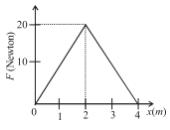Solution:

Area under curve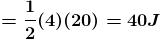W = work done by resistive force F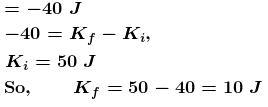*Answer can only contain numeric values
QUESTION: 2

### A particle moves with a velocity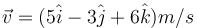under the influence of a constant force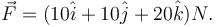The instantaneous power  (in Js–1) applied to the particle is :

Solution: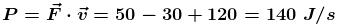*Answer can only contain numeric values
QUESTION: 3

### An electric motor creates a tension of 4500N in hoisting cable and reels it at the rate of  2m/s. What is the power of electric motor in kW?

Solution: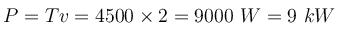*Answer can only contain numeric values
QUESTION: 4

A body of mass 5kg is acted upon by a variable force. The force varies with the distance covered by the body. Find the kinetic energy (in Joule) of the body when the body has covered 30m distance? Assume that the body starts from rest.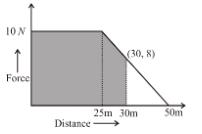Solution:

Work done by force when body has covered 25m
Area under curve upto distance 25m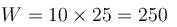Area between  25m to 30m is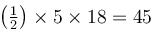So total work done by variable force till 30m is 250+45 = 295Joule
So, change in kinetic energy = 295J.
So final energy  = 295J.

*Answer can only contain numeric values
QUESTION: 5

In the figure shown initially spring is in unstretched state and blocks are at rest. Now 100N force is applied on block A and B  as shown in figure. After some time velocity of A becomes 2m/s and that of  4m/s and block A displaced by amount 10cm and spring is stretched by amount 30cm. Then work done by spring force on A will be (in Joule) :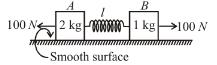Solution: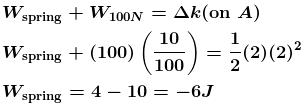*Answer can only contain numeric values
QUESTION: 6

The potential energy for a force field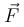is given by  U(x,y) = sin(y). The force acting on the particle of mass m at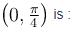Solution: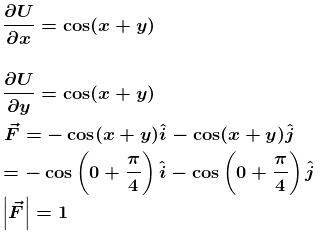*Answer can only contain numeric values
QUESTION: 7

A spring of force constant 800N/m has an extension of 5cm. The work done (in Joule) in extending it from 5cm to 15cm is :

Solution: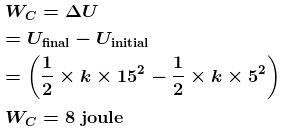*Answer can only contain numeric values
QUESTION: 8

The work done by the force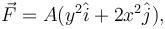where A is a constant and  x and y  in meters around the path shown is :

Take, A = 50N/m and d = 2.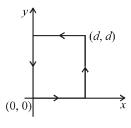Solution: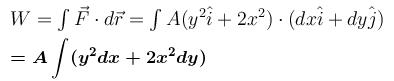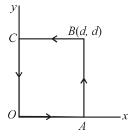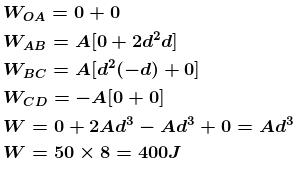*Answer can only contain numeric values
QUESTION: 9

An object is moving along a straight line path from P to Q under the action of a force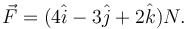If the co-ordinate of P and Q in metres are (3, 2, –1) and (2,-1,4) respectively. Then the work done by the force in Joule is :

Solution: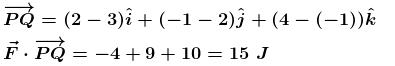*Answer can only contain numeric values
QUESTION: 10

Power supplied to a particle of mass 2kg varies with time as P =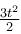watt. Here t is time in second. If velocity of particle at  t =0sec is v = 0m/s. The velocity of particle (in ms-1)  at time = 2sec  will be :

Solution:

Applying work energy theorem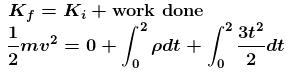Solving we get v = 2 m/s